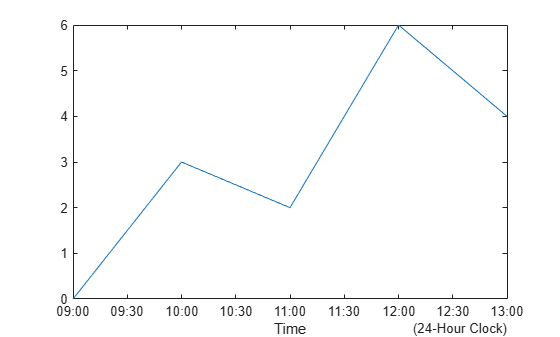# xsecondarylabel

Set x-axis secondary label

Since R2023b

## Syntax

``xsecondarylabel(label)``
``xsecondarylabel(Visible="off")``
``xsecondarylabel(Visible="on")``
``xsecondarylabel("auto")``
``xsecondarylabel(ax,___)``

## Description

example

````xsecondarylabel(label)` displays the specified text label as a secondary label on the x-axis of the current axes. If the x-axis already has secondary a label, the specified label replaces it.```

example

````xsecondarylabel(Visible="off")` hides the secondary label. You can specify the value of `Visible` as `false` or `0` to produce the same result. For example, `xsecondarylabel(Visible=0)` also hides the label.```
````xsecondarylabel(Visible="on")` displays the secondary label. You can specify the value of `Visible` as `true` or `1` to produce the same result. For example, `xsecondarylabel(Visible=true)` also displays the label. This syntax has no effect when the label is empty.```
````xsecondarylabel("auto")` resets the secondary label to its automatic state, which displays a secondary label in some cases. For example, a secondary label might automatically appear when you plot datetime values or very large numbers.```

example

````xsecondarylabel(ax,___)` modifies the secondary label for the target axes. Specify the target axes before any of the input argument combinations in the previous syntaxes.```

## Examples

collapse all

Plot a vector of numbers against a vector of time values. Then create an x-axis label, `"Time"`, and a secondary label, `"(24-Hour Clock)"`.

```x = datetime(2023,6,30,8,00,00,Format="HH:mm") + hours(1:5); y = [0 3 2 6 4]; plot(x,y); xlabel("Time") xsecondarylabel("(24-Hour Clock)")```Plot a vector of numbers against a vector of datetime values.

```x = datetime(2018,5,1:5); y = [3 1 6 4 10]; plot(x,y)```Hide the secondary label on the x-axis. The `"2018"` label disappears.

`xsecondarylabel(Visible="off")`Create two plots in a tiled chart layout. When you call the `nexttile` function, specify an output argument to get the axes object for later use.

```tiledlayout(2,1) % First plot ax1 = nexttile; x1 = datetime(2023,6,30,8,00,00,Format="HH:mm") + hours(1:5); y1 = 1:5; plot(x1,y1) % Second plot ax2 = nexttile; x2 = datetime(2023,6,30,13,00,00,Format="HH:mm") + hours(1:5); y2 = 5:-1:1; plot(x2,y2)```Change the secondary label for the x-axes in both plots by specifying the array `[ax1 ax2]` as the first argument to the `xsecondarylabel` function.

`xsecondarylabel([ax1 ax2],"(24-Hour Clock)")`## Input Arguments

collapse all

Label text, specified as a string scalar, string vector, character vector, or cell array of character vectors. To display one line of text, specify a string scalar or character vector. To specify multiple lines of text, specify a string vector or cell array of character vectors.

Example: `xsecondarylabel("Hurray for me!")`

Example: `xsecondarylabel('Hurray for you!')`

Target axes, specified as an `Axes` object or an array of `Axes` objects.

If you do not specify the target axes, then `xsecondarylabel` uses the current axes.

## Version History

Introduced in R2023b